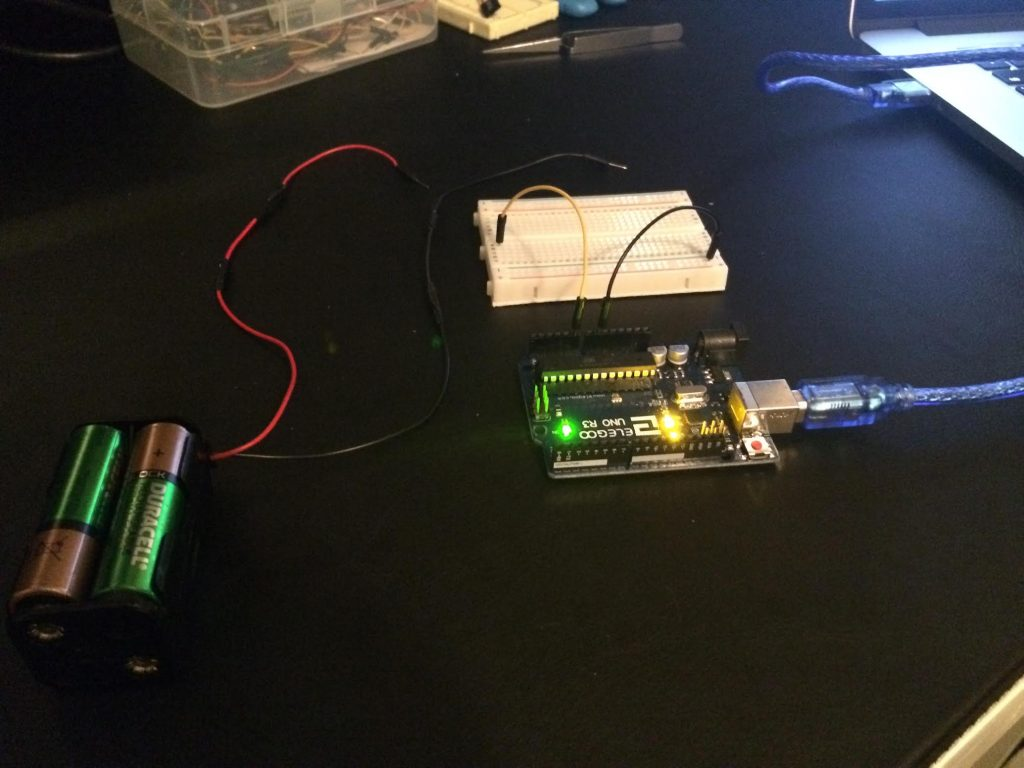# Arduino values to Python over Serial

I’ve done a little bit of reading on the ReadAnalogVoltage of Arduino’s home page, and they give a straight forward way to read voltage from an analog pin.

I wanted to take this one step further and send the value over serial, then read it in Python using pySerial.

My setup is very straight forward, I have a Arduino UNO, a bread board, and a battery pack holding 4x AA batteries:To start out I want to merely print the voltage value in Arduino Studio to the serial console, my code looks something like this:

```void setup() {
// connect to serial
Serial.begin(9600);
}

void loop() {

// read value from analog pin

// convert to voltage and print to serial connection
float voltage = sensorValue * ( 5.0 / 1023.0 );
Serial.println(voltage);

}
```

Now that we’ve verified this works, lets make a couple modification to the Arduino code.

Since the value of the analogRead may be over 255 (more than can fit in a single byte), we will need to send two bytes, a high byte, and a low byte. This concept is called most significant byte, and least significant byte.

```void setup() {
// connect to serial
Serial.begin(9600);
}

void loop() {

// read value from analog pin

// get the high and low byte from value
byte high = highByte(sensorValue);
byte low = lowByte(sensorValue);

// write the high and low byte to serial
Serial.write(high);
Serial.write(low);

}
```

Then on the Python side we can use pySerial to read two bytes, and convert using the formula Arduino gave us.

```import serial

# open our serial port at 9600 baud
dev = '/dev/cu.usbmodem1411'
with serial.Serial(dev, 9600, timeout=1) as ser:

while True:

# read 2 bytes from our serial connection

if raw:

# read the high and low byte
high, low = raw

# add up our bits from high and low byte
# to get the final value
val = ord(high) * 256 + ord(low)